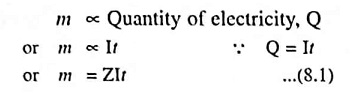## Faraday’s Laws of Electrolysis – First and Second Law:

The laws governing the electrolytic processes were formulated by Michael Faraday, an English Scientist, and are known as Faraday’s Laws of Electrolysis. These may be stated as below:

According to this law the chemical deposition due to flow of current through an electrolyte is directly proportional to the quantity of electricity (coulombs) passed through it.

i.e., mass of chemical deposition,where I is the steady current in amperes flowing through the electrolyte for t seconds and Z is a constant of proportionality and is known as the electrochemical equivalent of the substance.

If I = 1 A and t = 1 second, then Z = m

Thus electrochemical equivalent, Z, of a substance is defined as the amount of the substance deposited on passing a steady electric current of 1 A for one second through its solution. It is usually expressed in terms of milligrams per coulomb. The SI unit of electrochemical equivalent Z is the kilogram per coulomb (kg C-1).